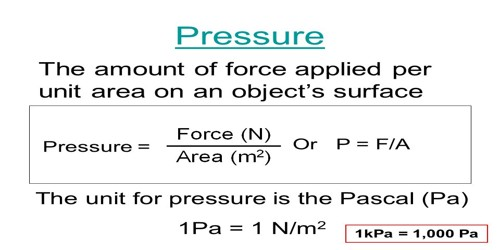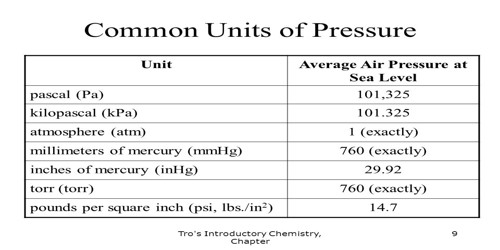# Unit of Pressure Explanation with Example

Unit of Pressure explanation with Example

Molecules in air randomly and continuously move to and fro and consequently force applied by these molecules per unit area on the container is called pressure of air. Since long atmosphere (abbreviated as atm) has been used as the unit of pressure, the amount of air pressure equal to the pressure of 760 mm mercury column on the sea level at 0°C and 45° latitude is called 1 atmosphere of pressure or (1 atm). In mm mercury mm Hg is also used as unit of pressure. In the name of famous scientist E. Torricelli the unit torr is also used as unit of pressure. That means 1 ton = 1 mm Hg. Nowadays for scientific experimental work pressure of gas in S.I. unit is expressed in the following way.

In S.I. unit pressure of gases is expressed as Newton/metre (Nm–2) or pascal (Pa). 1 Newton force acting per square metre is called 1 pascal.

1 Pa = 1 Nm-2

1 atmospheric pressure = 1 atm = 0.76 mHg pressure of mercury column

= 0.76 m x 13.5951 x 103 kg m-3 x 9.8 ms-2

= 1.013 x 105 Nm-2 = 101.325 kPa

1 atm = 1.013 x l05 Nm-2 = 101.32 kPa = 1.01 x 105 Pa = 760 mmHg.Due to change of temperature at a place capacity of containing particles of vapour also changes. But at a particular temperature, the capacity of containing particles of vapour remains fixed. It cannot accommodate extra vapour. In this condition pressure applied by vapour is called saturated vapour pressure.

Again, at a fixed temperature if the amount of vapour in a place is such that it can accommodate more vapour, then that vapour is called unsaturated vapour.

“Saturated vapour pressure at a place is 1.336 mm of mercur” means that at that particular place vapour will assert 1.336 mm mercury pressure.